Browse by Topic
Related Topics

# 16 Puzzles for International Puzzle Day

Today (Tuesday, January 29) is International Puzzle Day. To celebrate, here are 16 puzzles from the Wolfram Demonstrations Project.

 1. Box Packing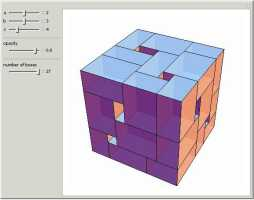2. Dissection Fallacy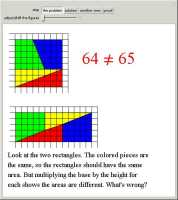Can 27 3×4×5 blocks be placed in a 12×12×12 box? How about 27 a×b×c blocks? Four identical shapes have an area of 64 or 65, depending on their arrangement. How? 3. Box Toppling Patterns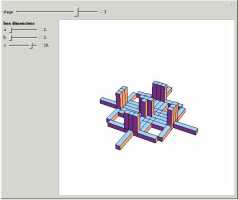4. Four-Color Maps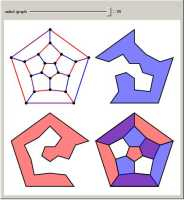A box gets rolled around on a floor. After 5 topples, how many different places can it be? What is the connection between borders and map coloring? 5. Shortest Time Problem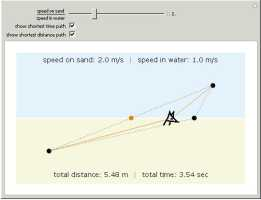6. Loculus of Archimedes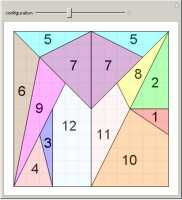A tennis ball is thrown in a lake. What route allows the ball to be retrieved in the shortest time? If a square is divided into the above shapes, how many different ways can the square be made? 7. Number of Squares in a Square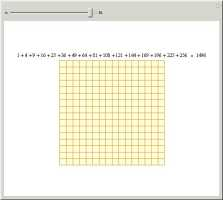8. Measuring the Speed of Light with Marshmallows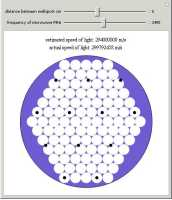How many squares are in this grid of squares? You have a bag of marshmallows and a microwave. How can you measure the speed of light? 9. The Statue of Regiomontanus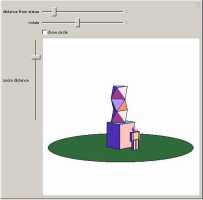10. Orchard-Planting Problem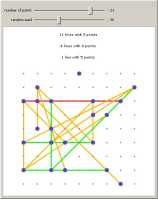Where should you stand so that a statue appears to be as large as possible? Can 10 trees be arranged so that there are 5 rows, each containing 4 trees? 11. Eight Queens Puzzle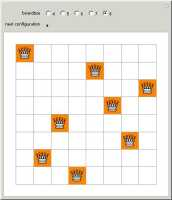12. Guilloché Patterns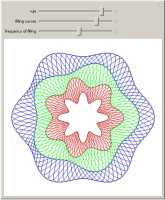How many ways can 8 queens be placed on a chessboard so that none attack each other? What are the rules for the strange curves found on paper currency? 13. Lights Out Puzzle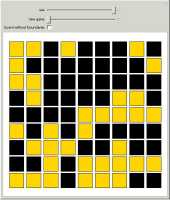14. Urn Problem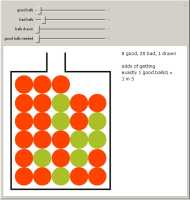Click on a square to change neighboring lights. How can all the lights be turned off? An urn holds 7 good balls, and 20 bad balls. If 5 balls are chosen, what are the odds that 2 will be good? 15. The Circle Covering Puzzle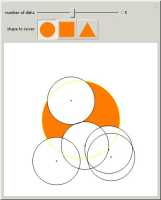16. Haberdasher’s Problem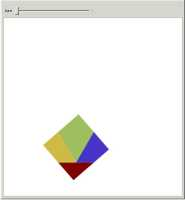Can you completely cover the orange shape with the given set of disks? Can a square be cut into 4 pieces and rearranged into an equilateral triangle?